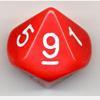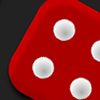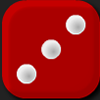# Resources tagged with: Rounding

Filter by: Content type:
Age range:
Challenge level:

There are 9 NRICH Mathematical resources connected to Rounding, you may find related items under The Number System and Place Value.

Broad Topics > The Number System and Place Value > Rounding### Reasoned Rounding

##### Age 7 to 14Challenge Level

Four strategy dice games to consolidate pupils' understanding of rounding.### Round the Dice Decimals 1

##### Age 7 to 11Challenge Level

Use two dice to generate two numbers with one decimal place. What happens when you round these numbers to the nearest whole number?### Round the Two Dice

##### Age 5 to 7Challenge Level

This activity focuses on rounding to the nearest 10.### Round the Dice Decimals 2

##### Age 7 to 11Challenge Level

What happens when you round these numbers to the nearest whole number?### Round the Four Dice

##### Age 7 to 11Challenge Level

This activity involves rounding four-digit numbers to the nearest thousand.### Rough Rectangle

##### Age 11 to 14 ShortChallenge Level

What is the smallest possible area that this rectangle could have?### Round the Three Dice

##### Age 7 to 11Challenge Level

What happens when you round these three-digit numbers to the nearest 100?### Archimedes and Numerical Roots

##### Age 14 to 16Challenge Level

The problem is how did Archimedes calculate the lengths of the sides of the polygons which needed him to be able to calculate square roots?### Route to Root

##### Age 16 to 18Challenge Level

A sequence of numbers x1, x2, x3, ... starts with x1 = 2, and, if you know any term xn, you can find the next term xn+1 using the formula: xn+1 = (xn + 3/xn)/2 . Calculate the first six terms of this. . . .